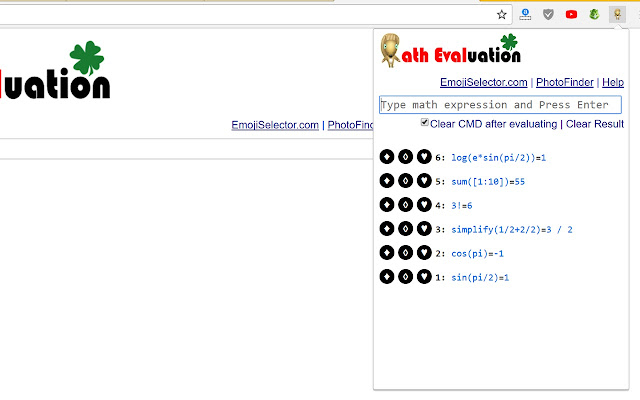# Math Evaluation (delisted)

CRX id

## ajllncehcekafimmgdilbcknonihefimDescription from extension meta

## This is a tool that allows you calculate the math expression and solve some advanced math problems & update search engine

Image from storeDescription from store As you know, math is very important but it's also difficult. This plugin helps you calculate the complex mathematical expression, solve some math problems. We hope this plugin can help you learn math easier and you no longer hate math. The functionalities of this plugin: 1) Calculate math expression. 2) Solve some math problems, such as solve equation, system equation, find intersection etc 3) Matrix calculation. 4) Other calculation. 5) new search Otherwise, you can copy the link of expression to send to your friends, share on facebook directly. This is very useful in math study. This version is limited 1 function - no display mathematical formula in graphical form.

### Latest reviews

• (2019-04-10) Habib Zaid: Contains browser hijacker initdex. dont install this
• (2017-12-28) Brian Upton: mathematical blessing
• (2017-03-10) Taddy Hork: very usefull
• (2017-03-10) Marry Queen: perfect on my iPhone
• (2017-03-10) Jack Danny: super stars

Installs
551 history
Category
Rating